Function Repository Resource:

# PermutationCyclesGraph

A graph representing cycles and fixed points of a permutation

Contributed by: Wolfram Staff
 ResourceFunction["PermutationCyclesGraph"][perm] constructs a graph of disjoint cycles representing the permutation perm.

## Details and Options

ResourceFunction["PermutationCyclesGraph"] accepts the same options as Graph.

## Examples

### Basic Examples (2)

Permutation cycles represented as a graph:

 In:=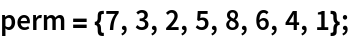In:=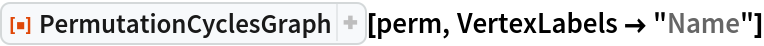Out=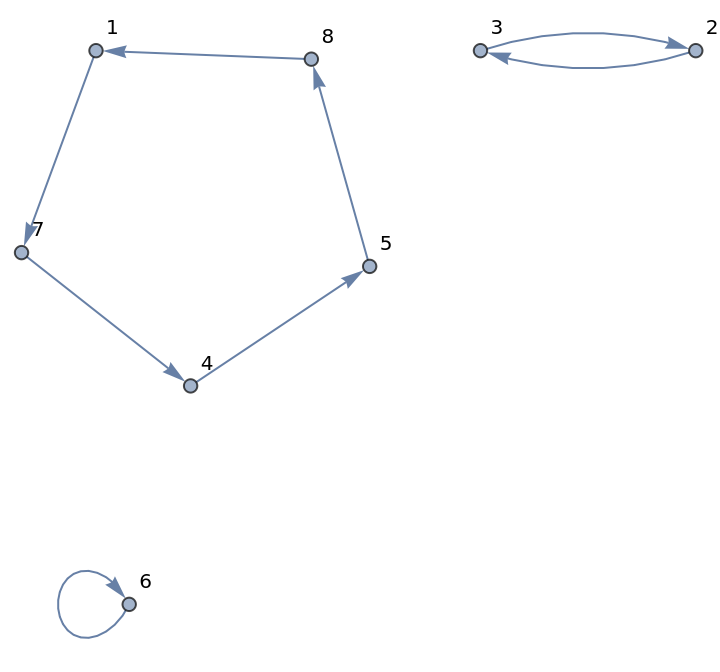Or as a list:

 In:=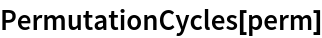Out=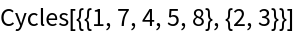### Scope (2)

Cycles graph of a permutation list:

 In:=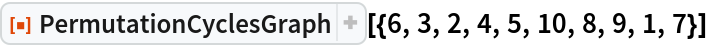Out=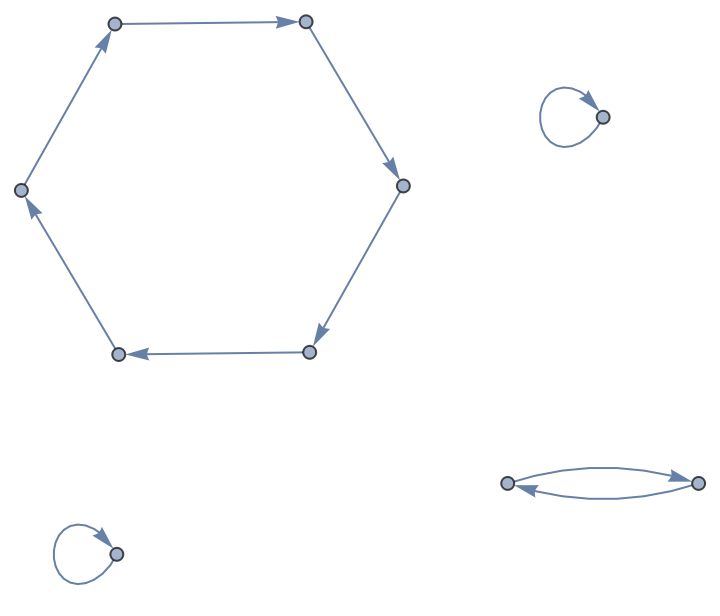Permutation represented as Cycles:

 In:=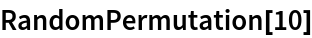Out=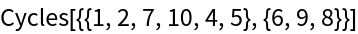In:=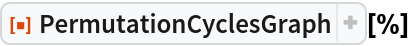Out=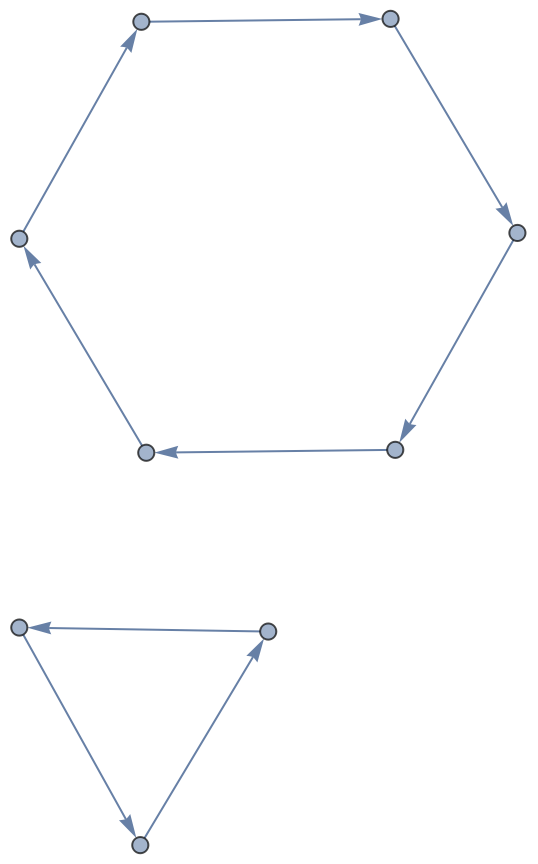### Properties and Relations (1)

Permutation cycles graph can also be constructed, possibly less efficiently, by finding the ordering of a permutation:

 In:=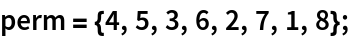In:=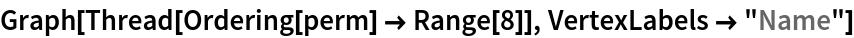Out=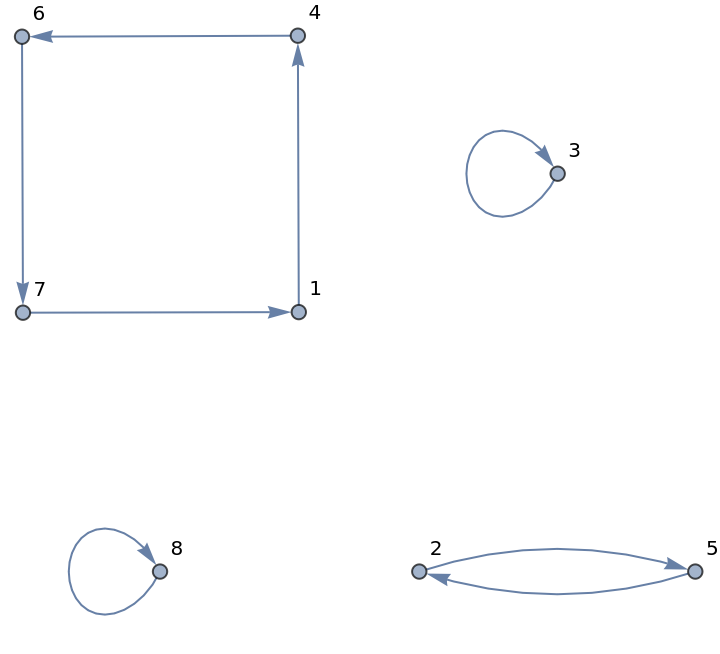In:=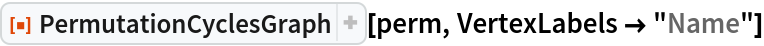Out=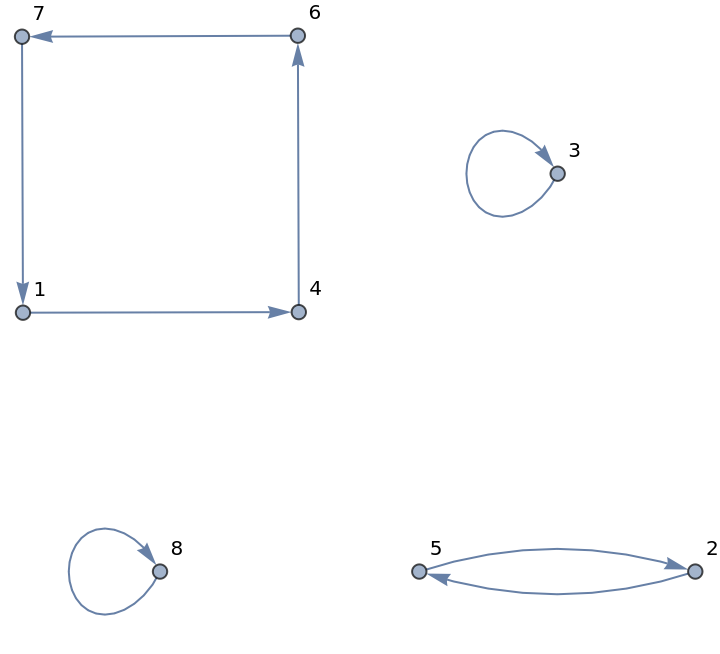### Possible Issues (3)

PermutationCyclesGraph of a permutation represented as cycles does not include fixed points; neither does the Cycles representation:

 In:=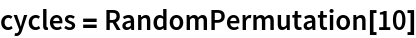Out=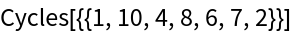In:=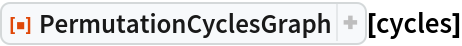Out=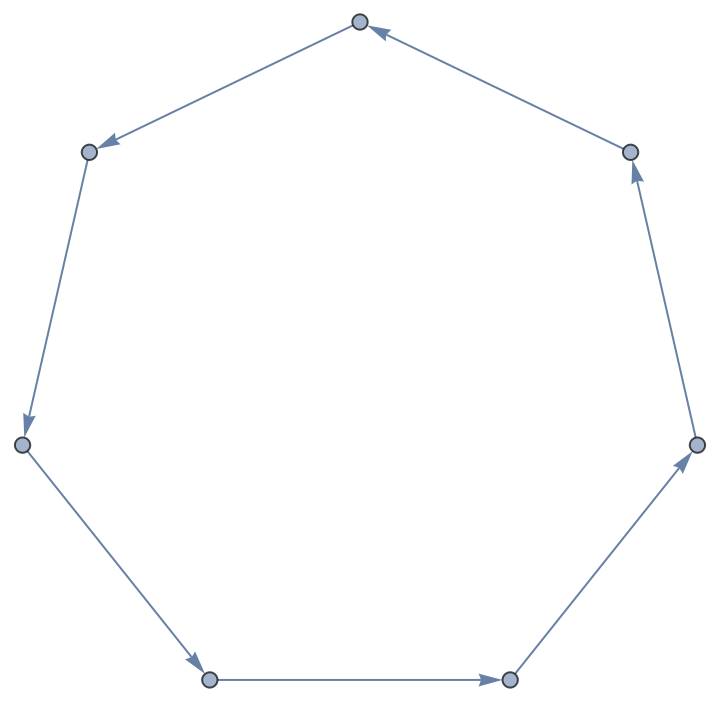Construct a permutation list to include fixed points:

 In:=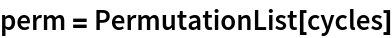Out=In:=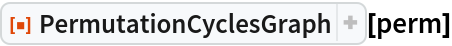Out=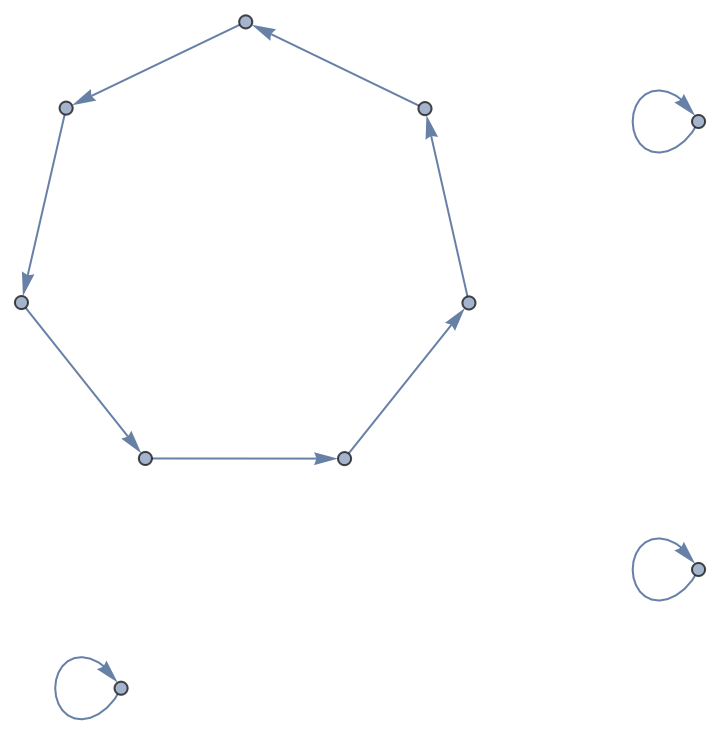Alternatively, use an arbitrary head, rather than Cycles, when computing permutation cycles:

 In:=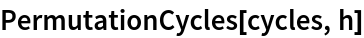Out=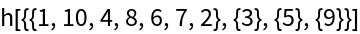In:=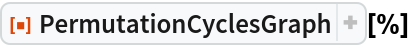Out=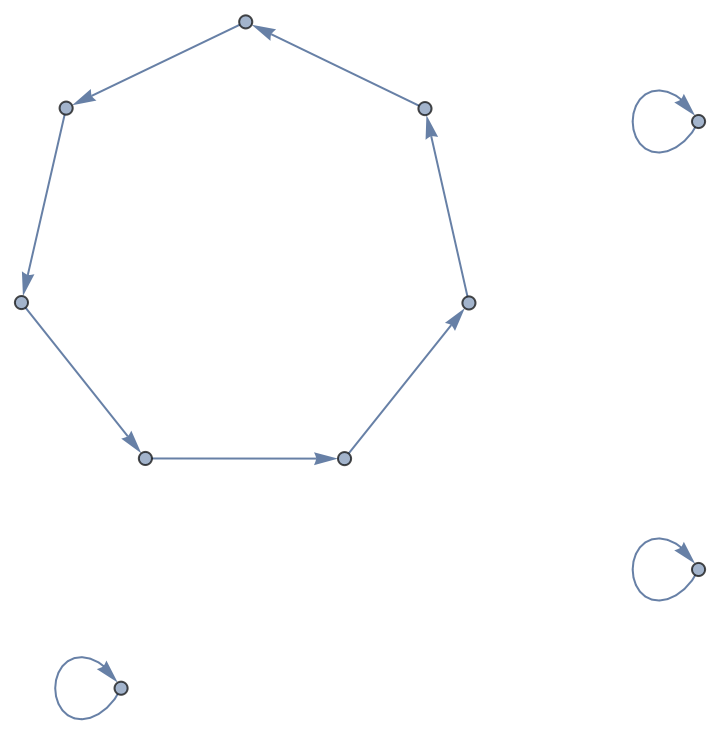## Version History

• 1.0.0 – 14 September 2020